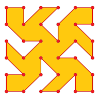# Resources tagged with: Making and proving conjectures

Filter by: Content type:
Age range:
Challenge level:

### There are 27 results

Broad Topics > Mathematical Thinking > Making and proving conjectures### DOTS Division

##### Age 14 to 16 Challenge Level:

Take any pair of two digit numbers x=ab and y=cd where, without loss of generality, ab > cd . Form two 4 digit numbers r=abcd and s=cdab and calculate: {r^2 - s^2} /{x^2 - y^2}.### Always a Multiple?

##### Age 11 to 14 Challenge Level:

Think of a two digit number, reverse the digits, and add the numbers together. Something special happens...### Regular Hexagon Loops

##### Age 11 to 14 Challenge Level:

Make some loops out of regular hexagons. What rules can you discover?### Multiplication Square

##### Age 14 to 16 Challenge Level:

Pick a square within a multiplication square and add the numbers on each diagonal. What do you notice?### Janine's Conjecture

##### Age 14 to 16 Challenge Level:

Janine noticed, while studying some cube numbers, that if you take three consecutive whole numbers and multiply them together and then add the middle number of the three, you get the middle number. . . .### Maxagon

##### Age 11 to 14 Challenge Level:

What's the greatest number of sides a polygon on a dotty grid could have?### Multiplication Arithmagons

##### Age 14 to 16 Challenge Level:

Can you find the values at the vertices when you know the values on the edges of these multiplication arithmagons?### What's Possible?

##### Age 14 to 16 Challenge Level:

Many numbers can be expressed as the difference of two perfect squares. What do you notice about the numbers you CANNOT make?### An Introduction to Magic Squares

##### Age 7 to 16##### Age 7 to 14 Challenge Level:

I added together some of my neighbours' house numbers. Can you explain the patterns I noticed?### Polycircles

##### Age 14 to 16 Challenge Level:

Show that for any triangle it is always possible to construct 3 touching circles with centres at the vertices. Is it possible to construct touching circles centred at the vertices of any polygon?### Dice, Routes and Pathways

##### Age 5 to 14

This article for teachers discusses examples of problems in which there is no obvious method but in which children can be encouraged to think deeply about the context and extend their ability to. . . .### Problem Solving, Using and Applying and Functional Mathematics

##### Age 5 to 18 Challenge Level:

Problem solving is at the heart of the NRICH site. All the problems give learners opportunities to learn, develop or use mathematical concepts and skills. Read here for more information.### A Little Light Thinking

##### Age 14 to 16 Challenge Level:

Here is a machine with four coloured lights. Can you make two lights switch on at once? Three lights? All four lights?### Close to Triangular

##### Age 14 to 16 Challenge Level:

Drawing a triangle is not always as easy as you might think!### Loopy

##### Age 14 to 16 Challenge Level:

Investigate sequences given by $a_n = \frac{1+a_{n-1}}{a_{n-2}}$ for different choices of the first two terms. Make a conjecture about the behaviour of these sequences. Can you prove your conjecture?### Consecutive Negative Numbers

##### Age 11 to 14 Challenge Level:

Do you notice anything about the solutions when you add and/or subtract consecutive negative numbers?### Triangles Within Pentagons

##### Age 14 to 16 Challenge Level:

Show that all pentagonal numbers are one third of a triangular number.### Triangles Within Squares

##### Age 14 to 16 Challenge Level:

Can you find a rule which relates triangular numbers to square numbers?### Helen's Conjecture

##### Age 11 to 14 Challenge Level:

Helen made the conjecture that "every multiple of six has more factors than the two numbers either side of it". Is this conjecture true?### Triangles Within Triangles

##### Age 14 to 16 Challenge Level:

Can you find a rule which connects consecutive triangular numbers?##### Age 14 to 16 Challenge Level:### To Prove or Not to Prove

##### Age 14 to 18

A serious but easily readable discussion of proof in mathematics with some amusing stories and some interesting examples.### Curvy Areas

##### Age 14 to 16 Challenge Level:

Have a go at creating these images based on circles. What do you notice about the areas of the different sections?### How Old Am I?

##### Age 14 to 16 Challenge Level:

In 15 years' time my age will be the square of my age 15 years ago. Can you work out my age, and when I had other special birthdays?